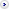Last visit was: Sun Jan 23, 2022 5:14 am It is currently Sun Jan 23, 2022 5:14 am

 Page 6 of 6 [ 77 posts ] Go to page Previous  1, 2, 3, 4, 5, 6
Porting the C64 compiler to target OPC5 (or OPC6)
Author Message

Joined: Sat Feb 02, 2013 9:40 am
Posts: 1576Re: Porting the C64 compiler to target OPC5 (or OPC6)
Not sure if this affects OPC5 or not, but I put a fix in.

Found an error in the C compiler which caused tabular based switch case statements to be optimized away. If the case values are dense enough the compiler will use a jump table rather than a series of conditional tests. But it puts all the case target labels out as data rather than code labels so the optimizer couldn't find the labels, and hence optimized them out, then it optimized out the code because it was unreachable.

This was found while working on the compiler for FT64.

A case statement like:
Code:
switch(spriteno)
{
case 0: x = 128; y = 32; break;
case 1: x = 160; y = 32; break;
case 2: x = 192; y = 32; break;
case 3: x = 224; y = 32; break;
case 4: x = 256; y = 32; break;
case 5: x = 288; y = 32; break;
case 6: x = 320; y = 32; break;
case 7: x = 352; y = 32; break;
case 8: x = 384; y = 32; break;
case 9: x = 416; y = 32; break;
case 10: x = 448; y = 64; break;
case 11: x = 480; y = 64; break;
case 12: x = 128; y = 64; break;
case 13: x = 160; y = 64; break;
case 14: x = 192; y = 64; break;
case 15: x = 224; y = 64; break;
case 16: x = 256; y = 64; break;
case 17: x = 288; y = 64; break;
case 18: x = 320; y = 64; break;
case 19: x = 352; y = 64; break;
case 20: x = 384; y = 64; break;
case 21: x = 416; y = 64; break;
case 22: x = 448; y = 64; break;
case 23: x = 480; y = 64; break;
case 24: x = 128; y = 96; break;
case 25: x = 160; y = 96; break;
case 26: x = 192; y = 96; break;
case 27: x = 224; y = 96; break;
case 28: x = 256; y = 96; break;
case 29: x = 288; y = 96; break;
case 30: x = 320; y = 96; break;
case 31: x = 352; y = 96; break;
}

got all optimized away.

_________________
Robert Finch http://www.finitron.ca

Wed Dec 27, 2017 6:35 amJoined: Wed Jan 09, 2013 6:54 pm
Posts: 1666Re: Porting the C64 compiler to target OPC5 (or OPC6)
Good catch! It's impressive that it was able to erase the evidence, that says something good about the optimiser.

Wed Dec 27, 2017 8:33 am
 Page 6 of 6 [ 77 posts ] Go to page Previous  1, 2, 3, 4, 5, 6

Who is online

Users browsing this forum: CCBot and 0 guests

 You cannot post new topics in this forumYou cannot reply to topics in this forumYou cannot edit your posts in this forumYou cannot delete your posts in this forumYou cannot post attachments in this forum

 Jump to:  Select a forum ------------------ General Discussions Newbies Software    General programming    Languages and tools    Kernels and operating systems Hardware    Hardware in general    CPU/MCU choices and designs    Implementation and Construction Programmable logic Simulation and emulation Nostalgia Projects Anycpu.org